# Friday, March 24th

John Denver-Today (live).mp3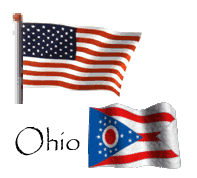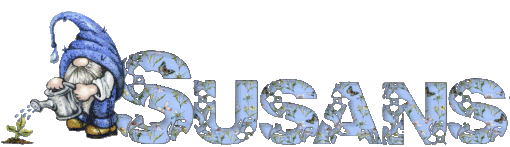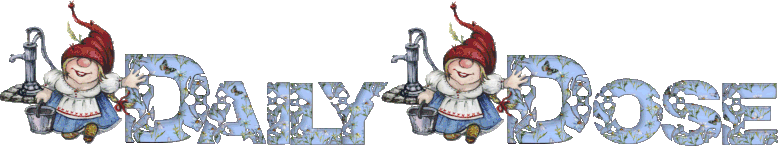Susan Kistler, Creator-Publisher
Since 2001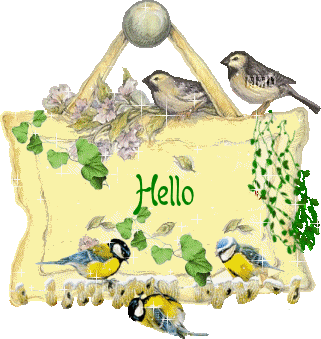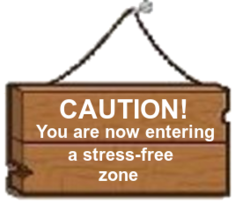Yes, things end,

but memories

last forever ....

Hold tight

to those ...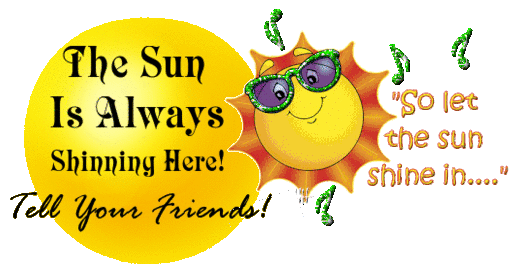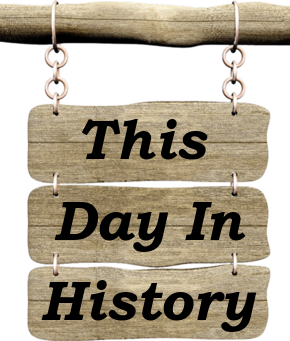In 1989 the oil tanker Exxon Valdez ran aground,
spilling some 11 million gallons [41 million litres]
of oil into Prince William Sound in Alaska and
creating the largest oil spill in U.S.
history up to that time.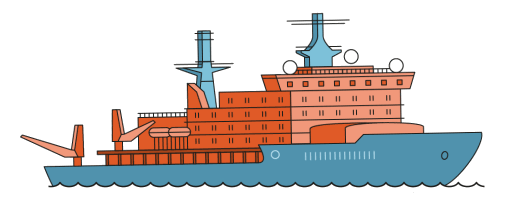In 1964 the half-dollar coin (or fifty cent piece) with
John F Kennedy on it was officially released to the
public. It was intended to be a memorial to the late
President who was assassinated 5 months earlier.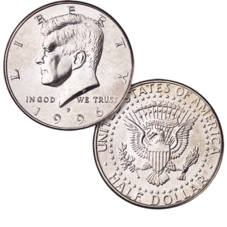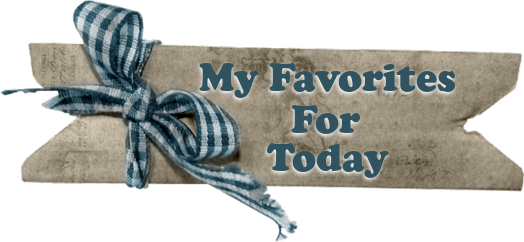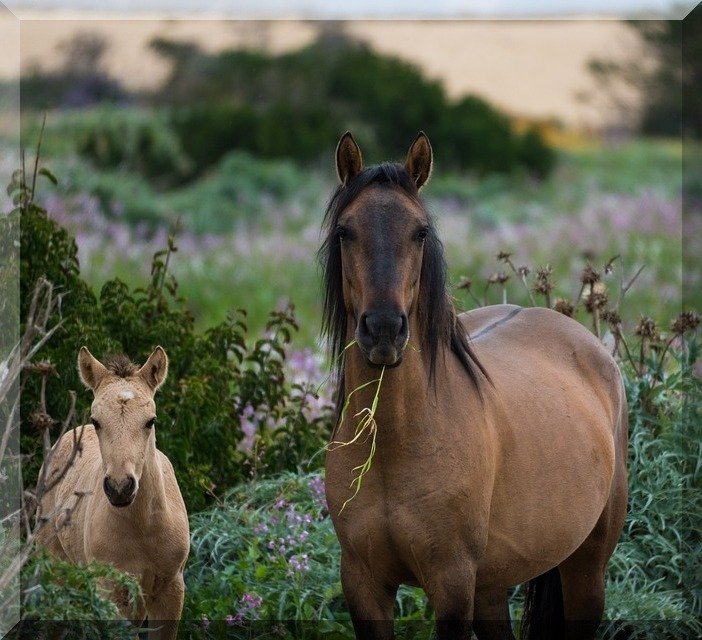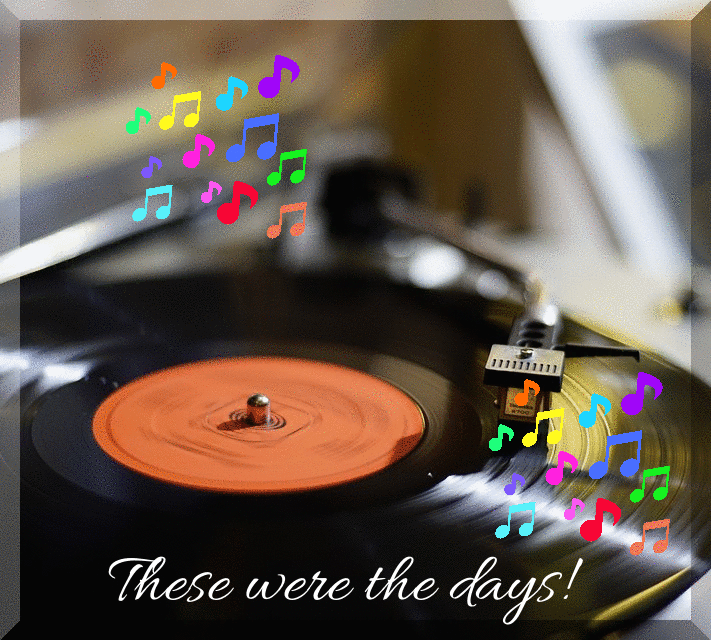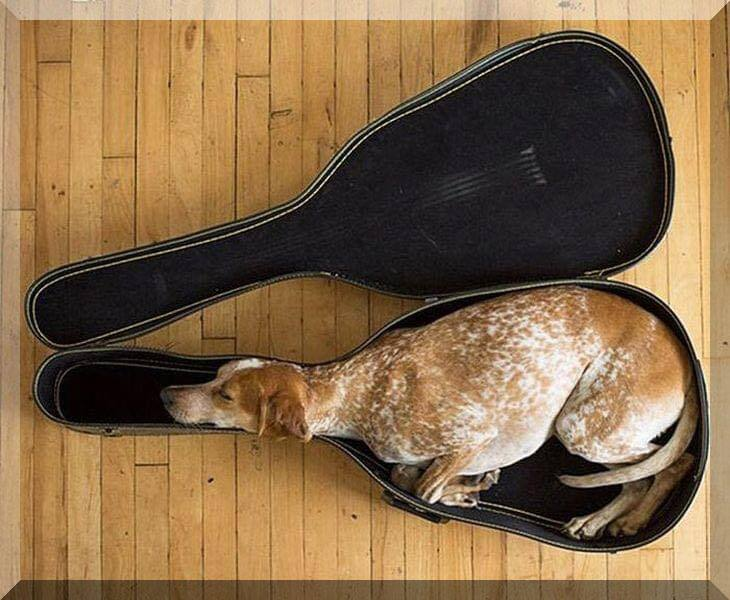I fit!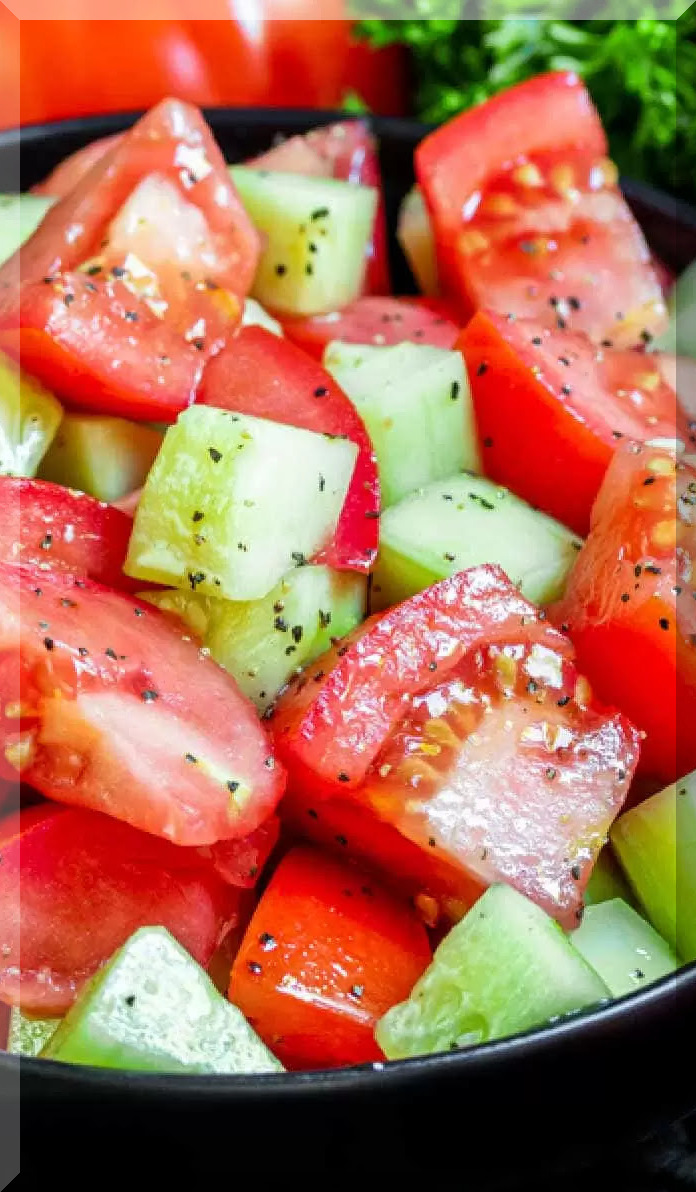Summer goodness on it's way!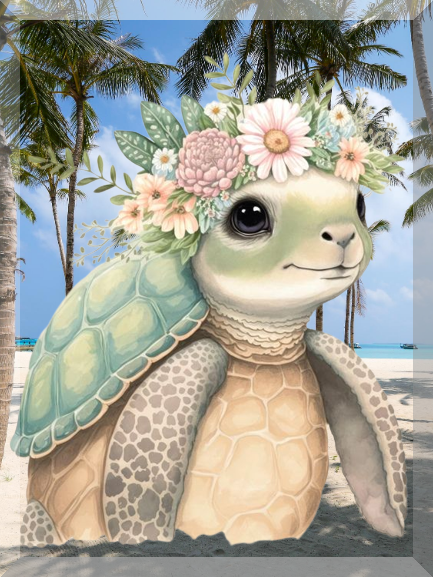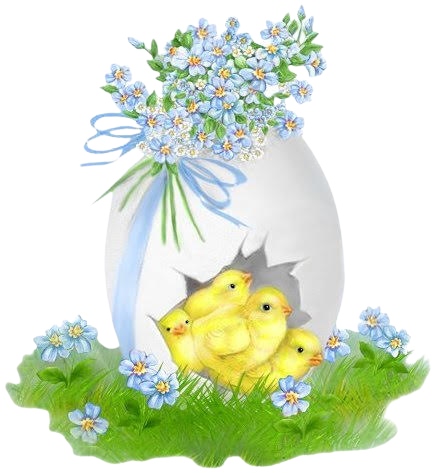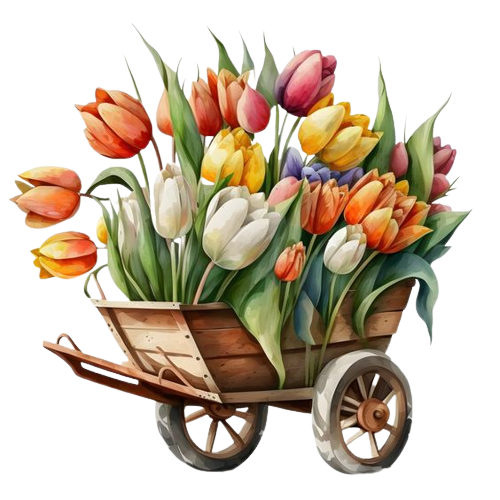^^^^^^^^^^^^^^^^^^^^^^^^^^^^^^^^^^^^^^^^^^^^^^^^^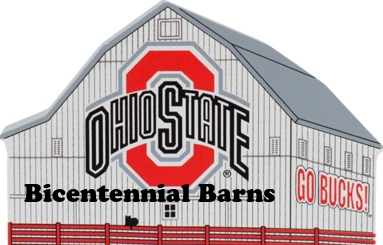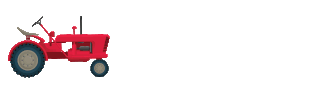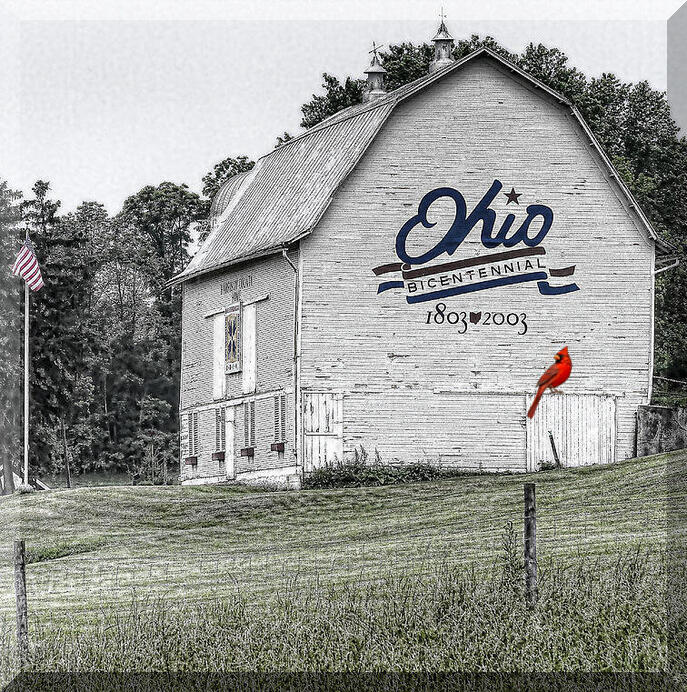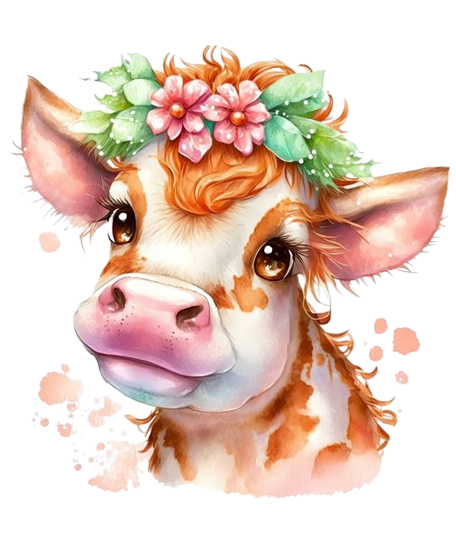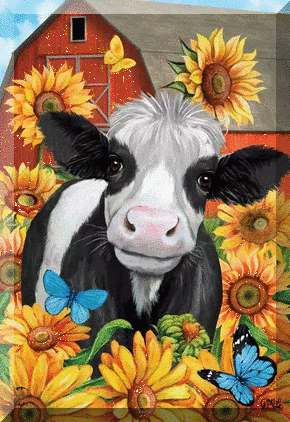^^^^^^^^^^^^^^^^^^^^^^^^^^^^^^^^^^^^^^^^^^^^^^^^^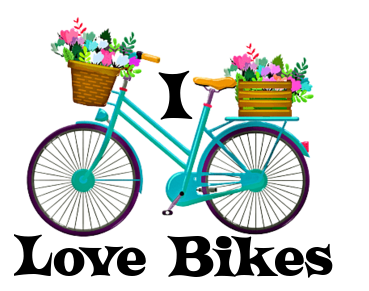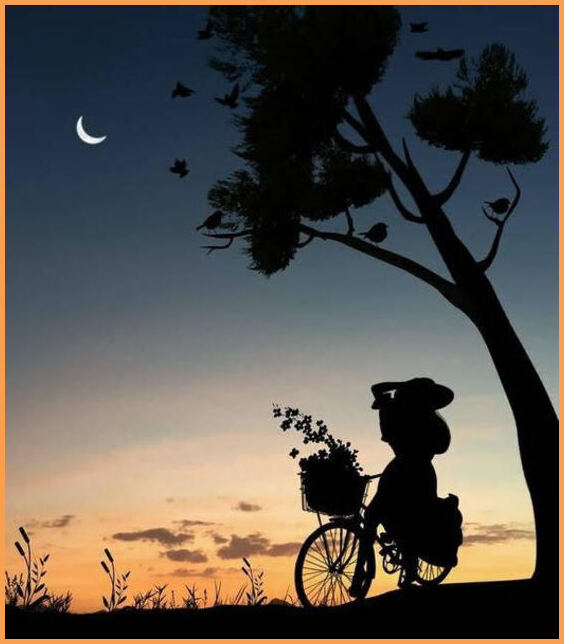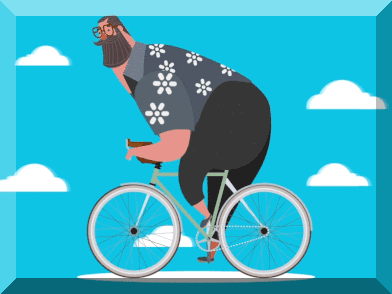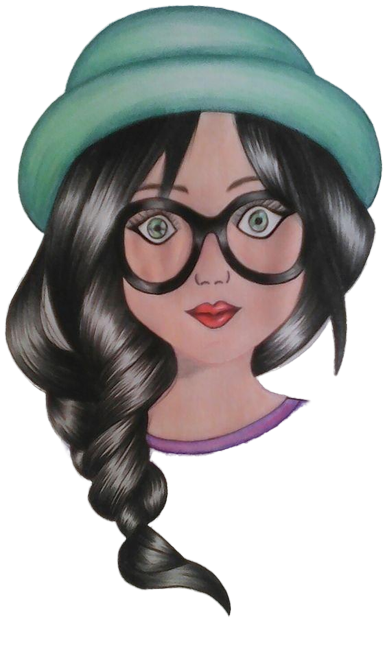^^^^^^^^^^^^^^^^^^^^^^^^^^^^^^^^^^^^^^^^^^^^^^^^^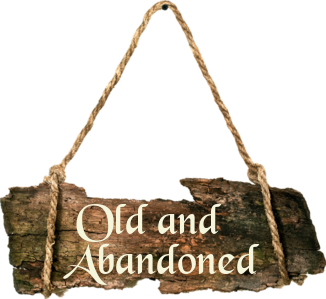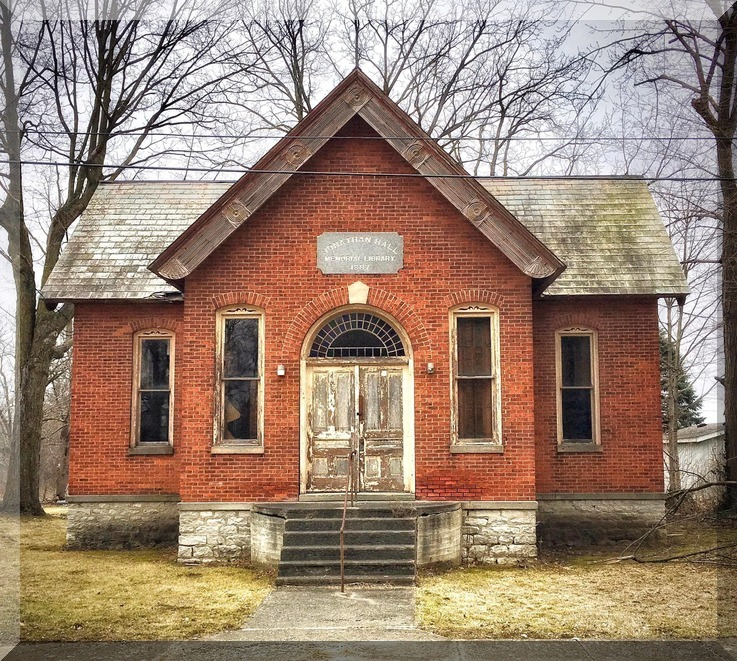If only these walls could talk. The teachers
and kids could probably tell us stories
of the good ole days.

^^^^^^^^^^^^^^^^^^^^^^^^^^^^^^^^^^^^^^^^^^^^^^^^^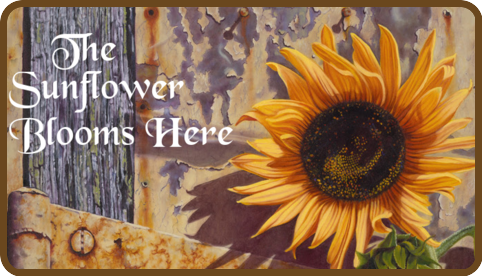Step by step, the sunflower
will open for me and you.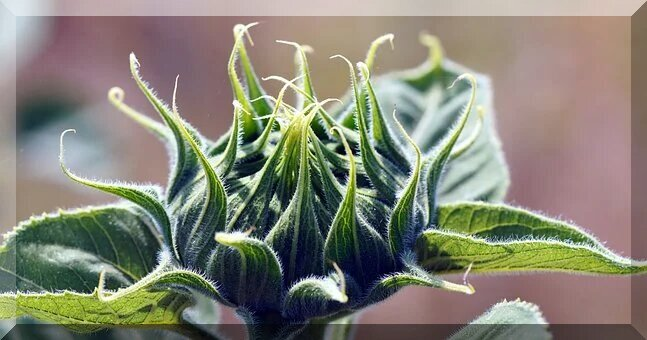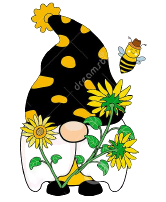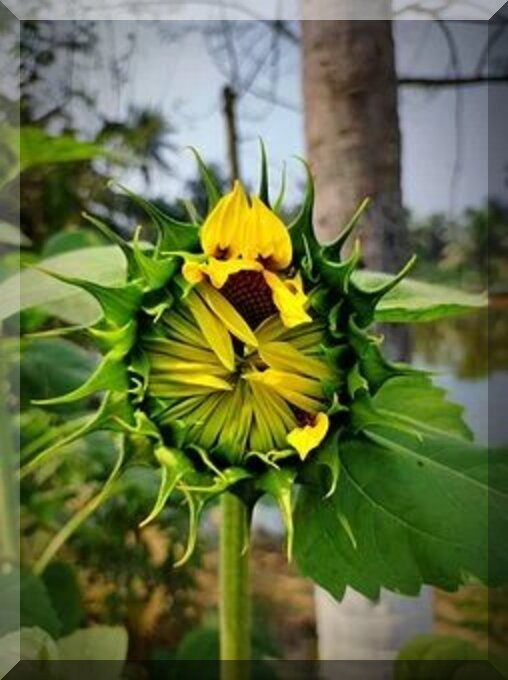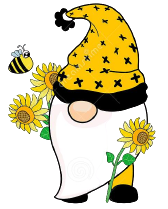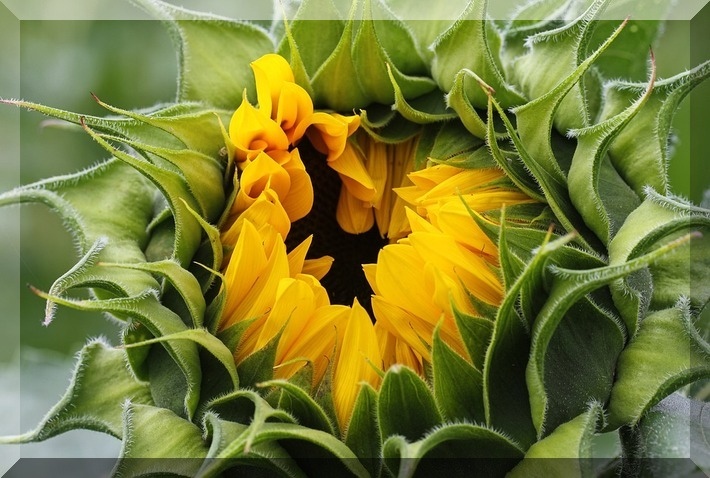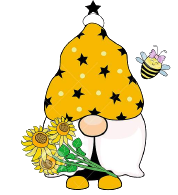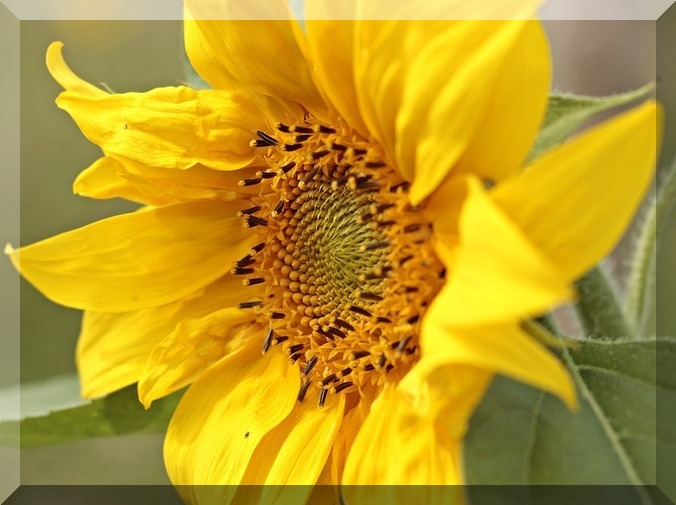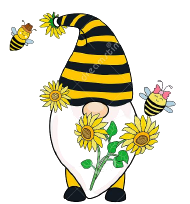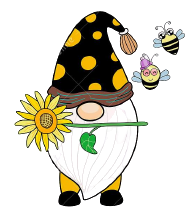^^^^^^^^^^^^^^^^^^^^^^^^^^^^^^^^^^^^^^^^^^^^^^^^^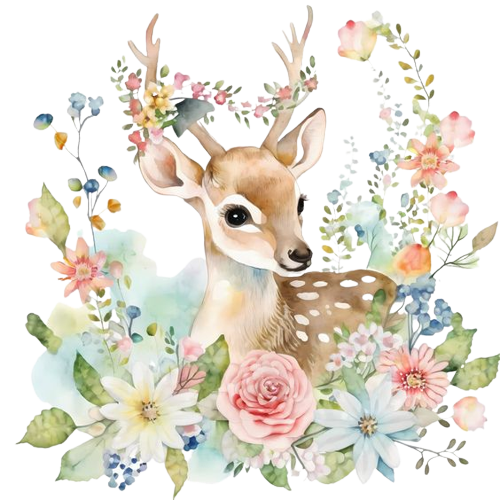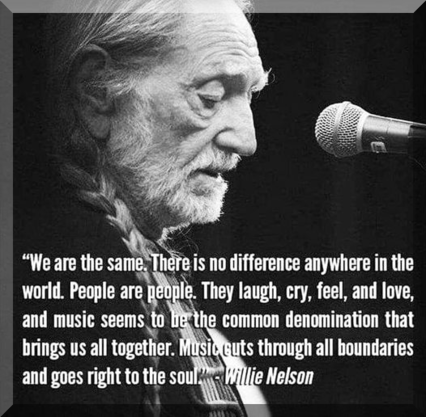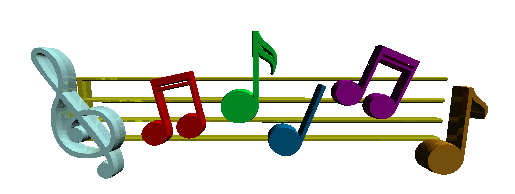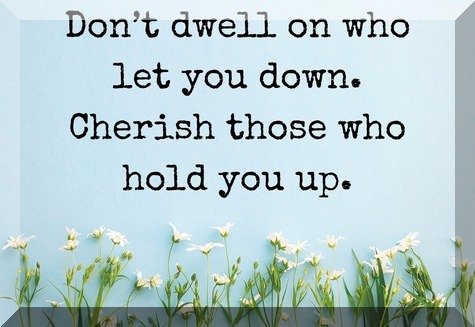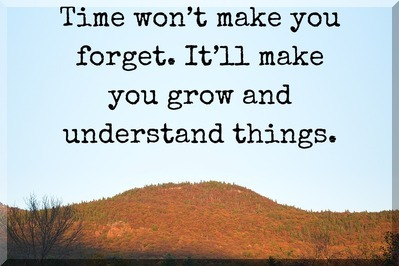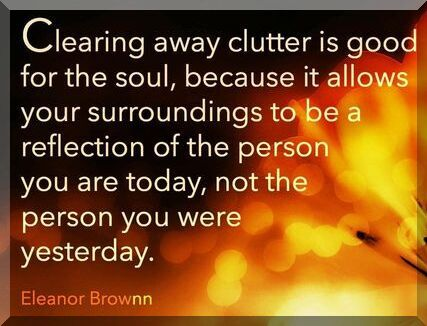^^^^^^^^^^^^^^^^^^^^^^^^^^^^^^^^^^^^^^^^^^^^^^^^^

Let's throw in some
bacon today!!
Wrap away ....

Green beans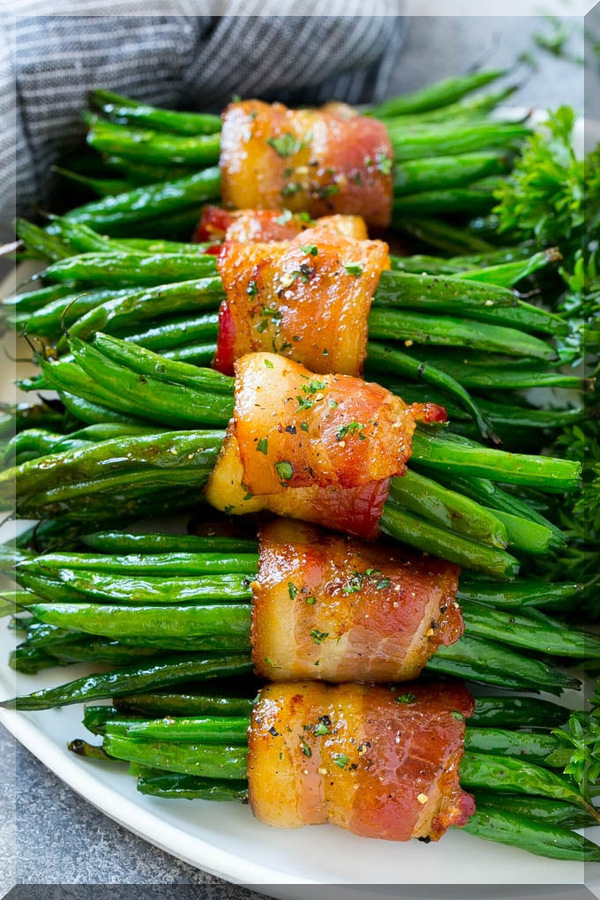Lil' SausagesFilet Mignon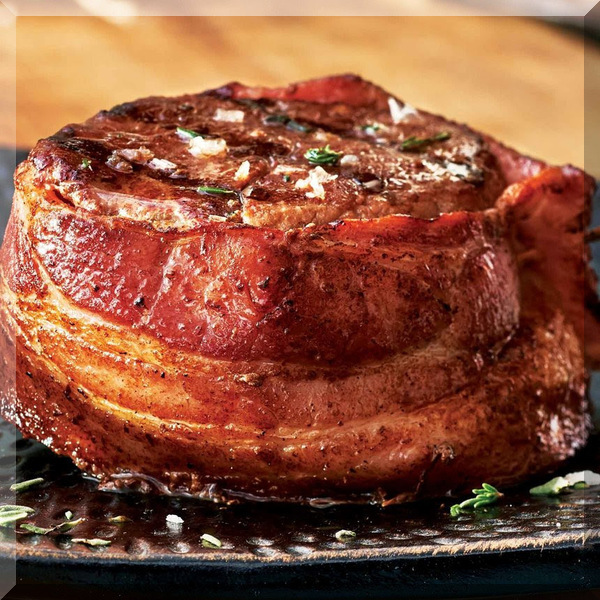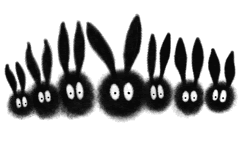^^^^^^^^^^^^^^^^^^^^^^^^^^^^^^^^^^^^^^^^^^^^^^^^^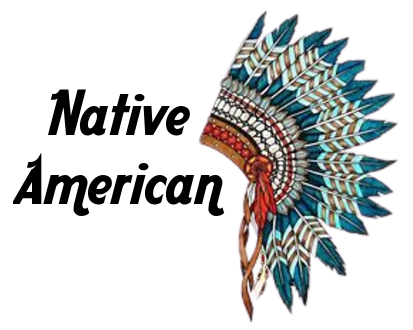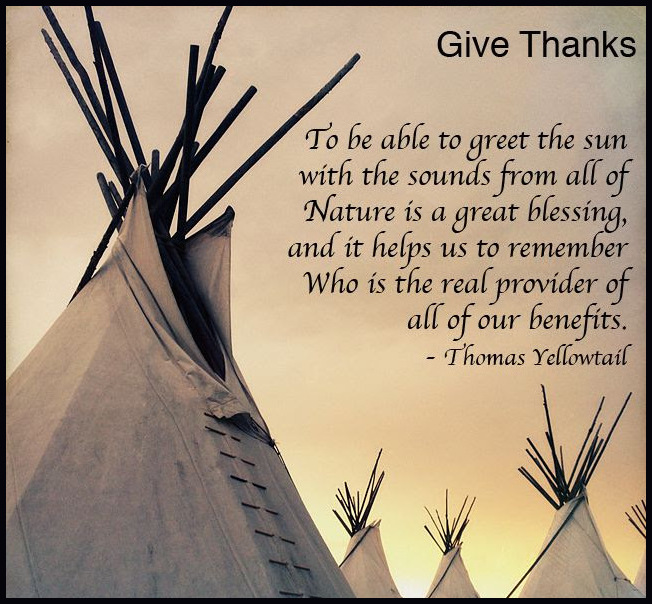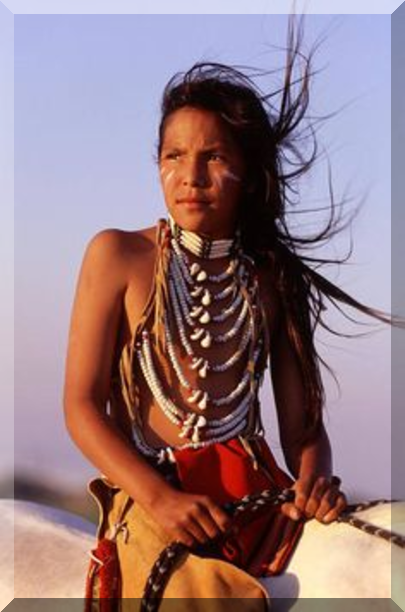^^^^^^^^^^^^^^^^^^^^^^^^^^^^^^^^^^^^^^^^^^^^^^^^^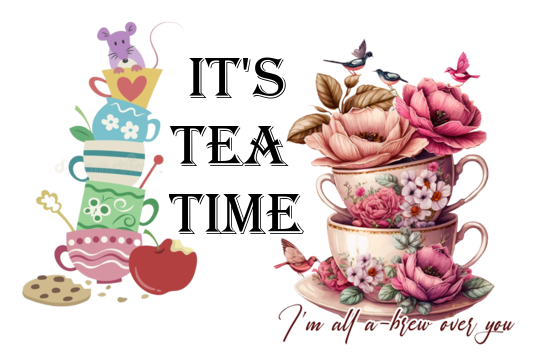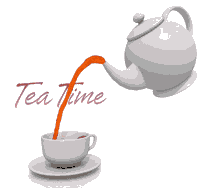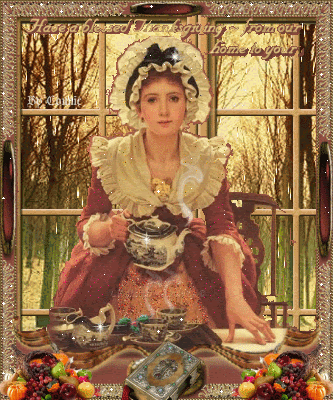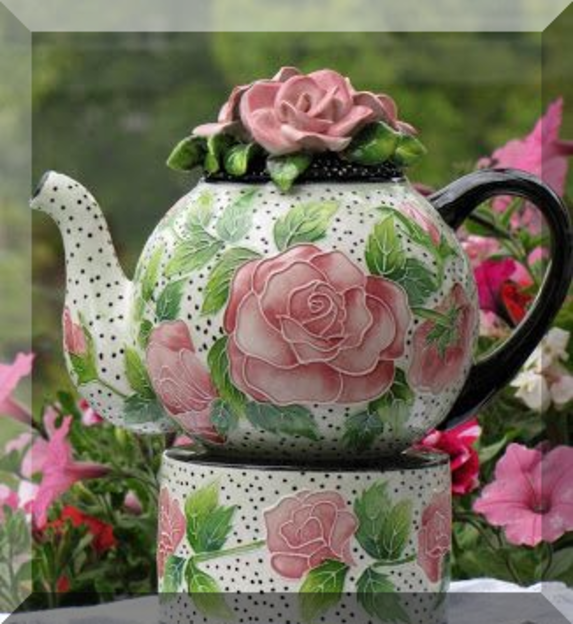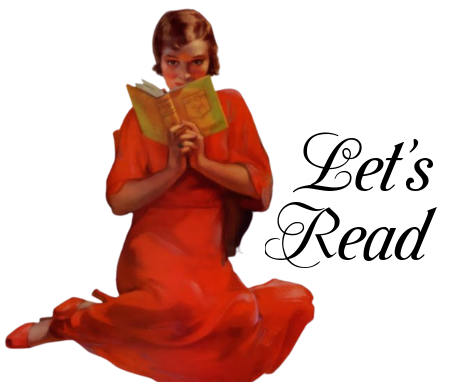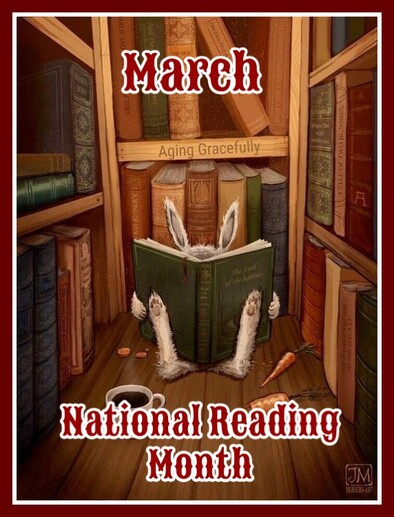In honor of Dr Suess' birthday, March is
designated as Nation Reading Month - a month
to motivate Americans of all ages to read every
day. Reading is fun and has many benefits,
regardless of your age. It's a key component
of education and professional development.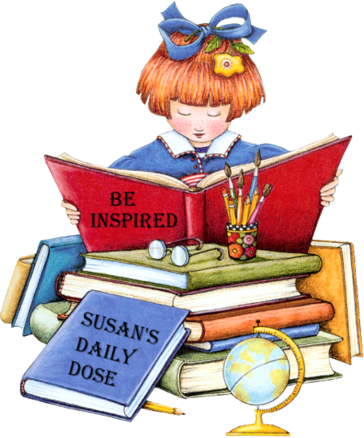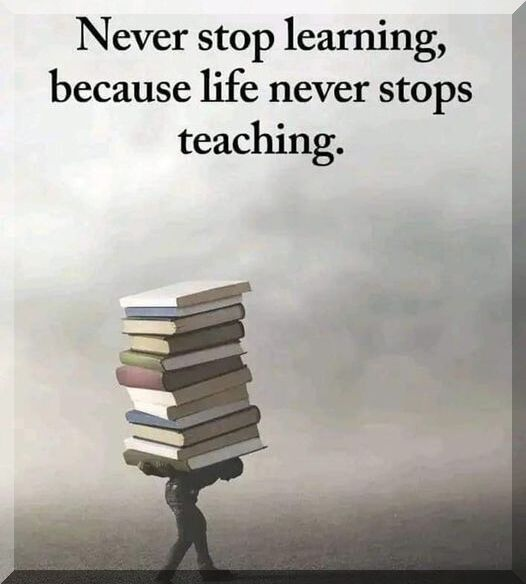^^^^^^^^^^^^^^^^^^^^^^^^^^^^^^^^^^^^^^^^^^^^^^^^^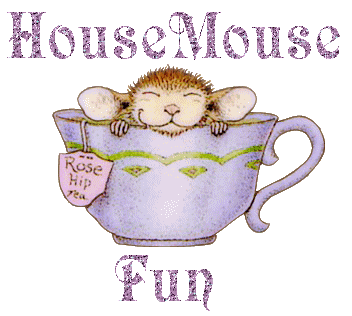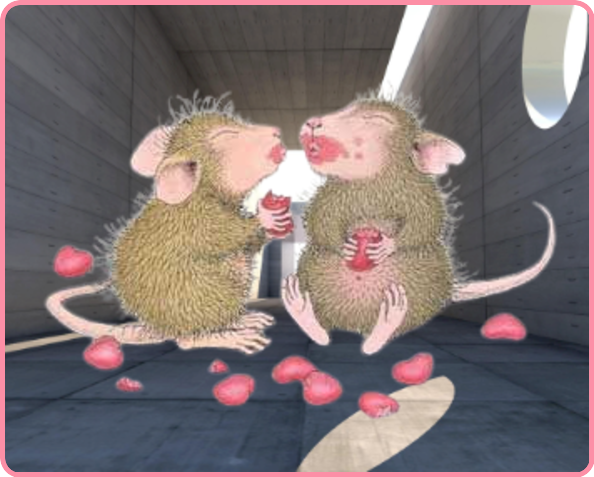^^^^^^^^^^^^^^^^^^^^^^^^^^^^^^^^^^^^^^^^^^^^^^^^^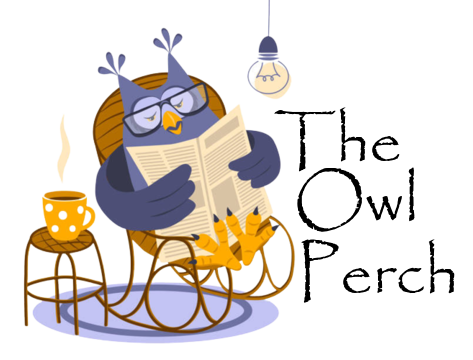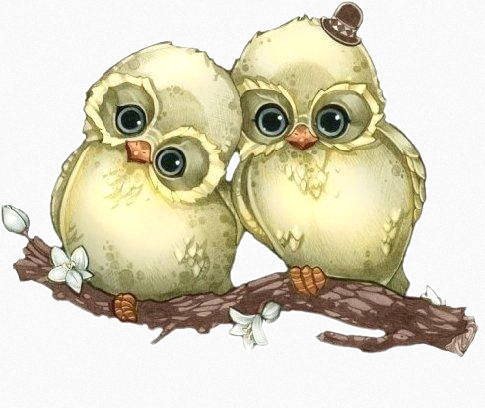^^^^^^^^^^^^^^^^^^^^^^^^^^^^^^^^^^^^^^^^^^^^^^^^^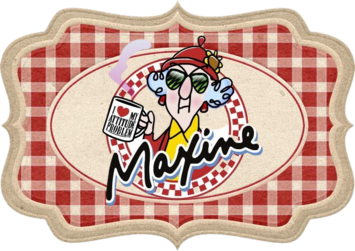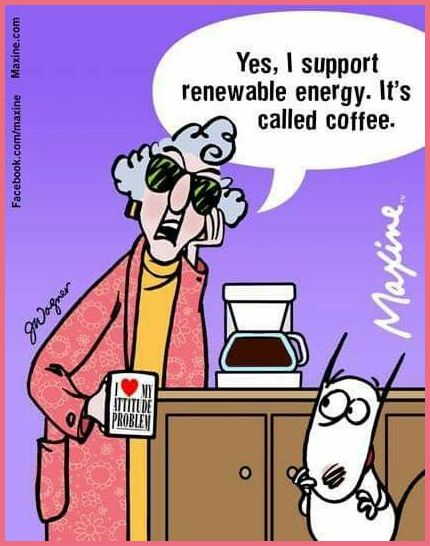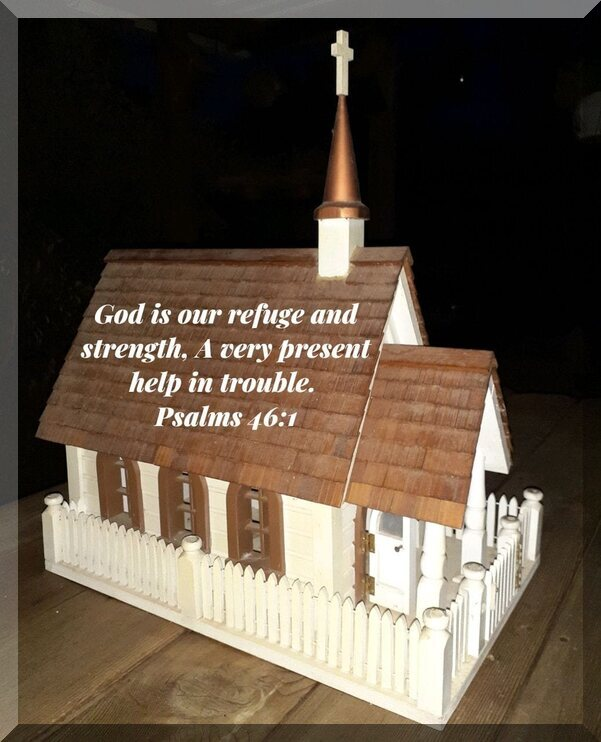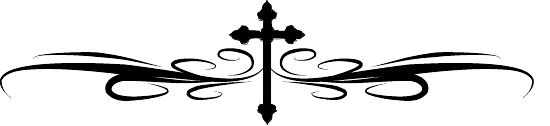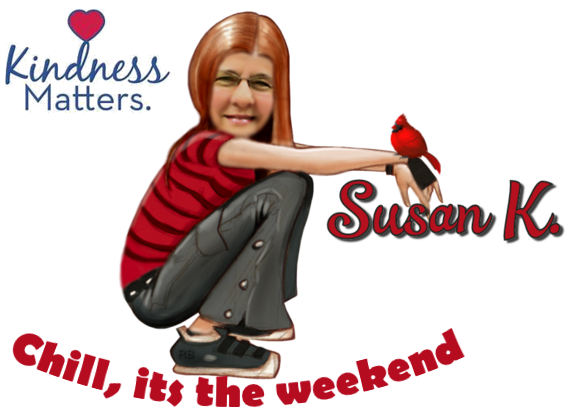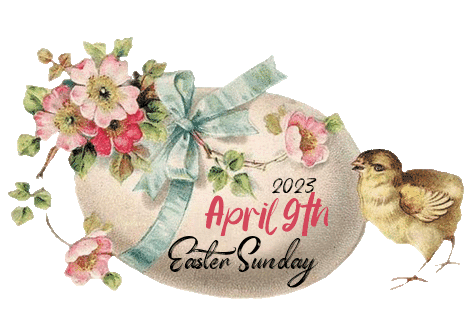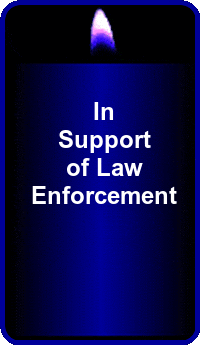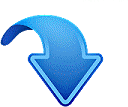I have taken time to include on my pages below.
I will appreciate if you do!
Thank you.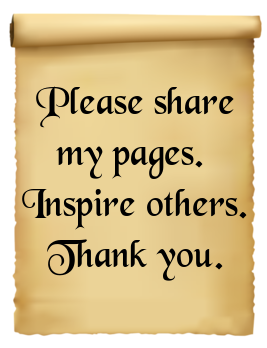Sharing a wonderful blog/website
https://tattysthingies.blogspot.com/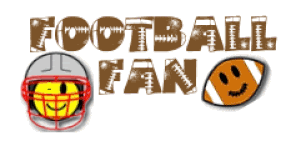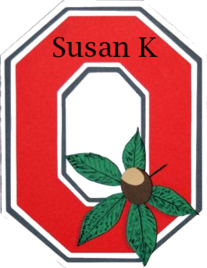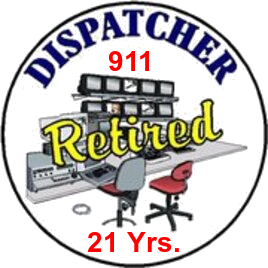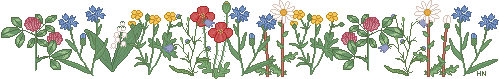Hello, and I would like to let you know that I do accept
donations to help pay for this website. Donations do help
me a lot and are very appreciated. The donate button above
will lead you to PayPal, a safe and easy way to donate.
Not comfortable with PayPal, simply email me and I can
send you my address for mailing. Thank you.The below site will help animals and others. Please
do visit and see how you can help by "just clicking"!
It only takes a minute. I used to have this on my
pages and quit for one reason or another and I
very much wanted to do it again. We all CAN make
a difference. VISIT TODAY!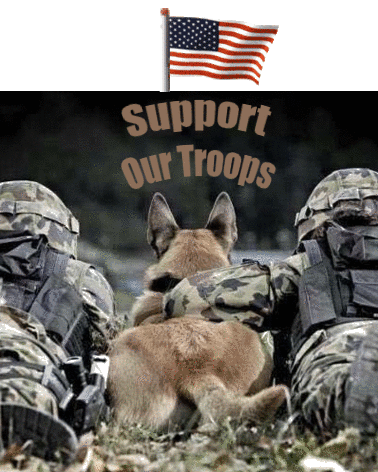The below map shows
location of visitors